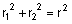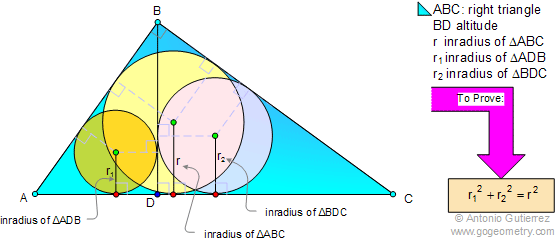# Geometry Problem 24 with Solution: Problem 24 with Solution: Right triangle, Altitude, Incircles.

< PREVIOUS PROBLEM  |  NEXT PROBLEM >

In triangle ABC, angle B is a right angle. BD is the altitude, r, r1, and r2, are the inradii of the triangles ABC, ADB, and BDC respectively. Prove that.Home | Geometry | Altitude | Problems | Open Problems | 10 Problems | 21-30 | Circle Tangent Line | Right triangle, Altitude and Incircle | Email |  | By Antonio Gutierrez
Last updated: Sep 19, 2014

Post or view a solution to the problem 24